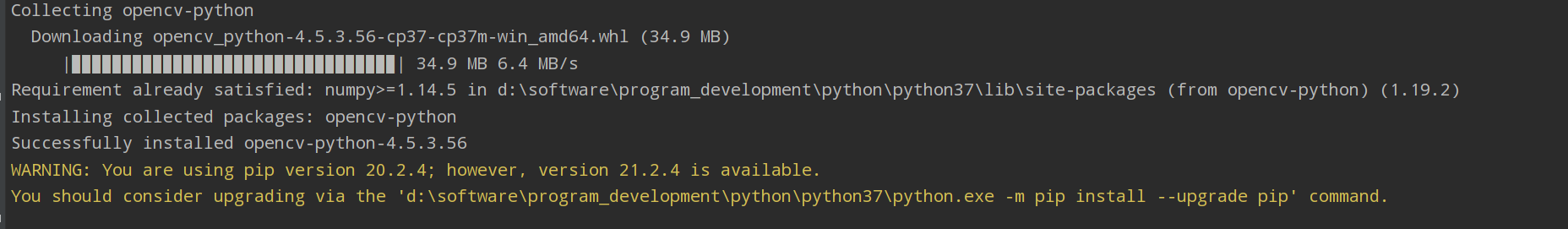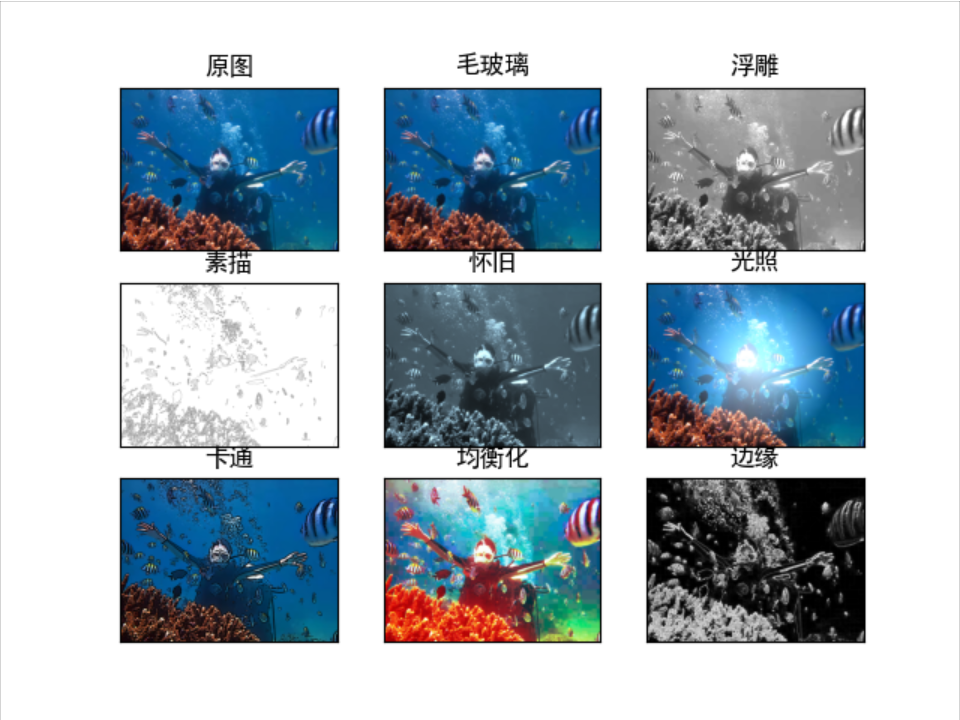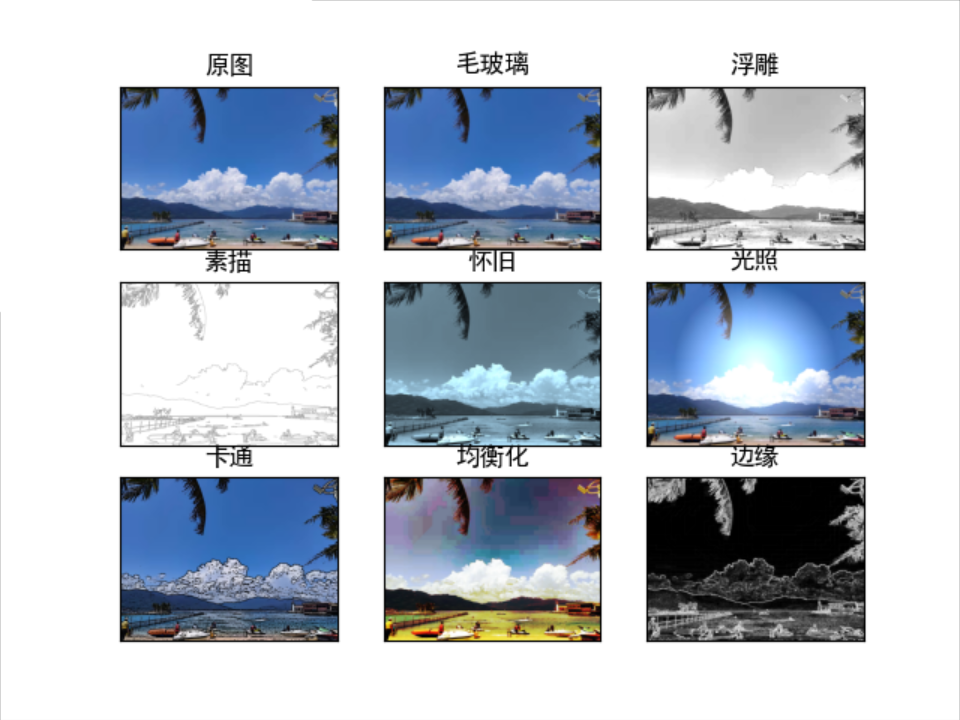`OpenCV` 是一个基于BSD许可（开源）发行的跨平台计算机视觉库，可以运行在Linux、Windows、Android和Mac OS操作系统上。它轻量级而且高效——由一系列 C 函数和少量 C++ 类构成，同时提供了Python、Ruby、MATLAB等语言的接口，实现了图像处理和计算机视觉方面的很多通用算法。

`OpenCV-Python` 是 OpenCV 的 Python 的 API 接口，它拥有 OpenCV C++ API 的功能，同时也拥有 Python 语言的特性,可以做到跨平台使用。### 环境搭建

 `1234` ``````import cv2 import numpy as np import matplotlib.pyplot as plt import math ``````

 `12` ``````pip install opencv-python pip install matplotlib ``````### 专属头像制作

 `123456789101112131415161718192021222324252627282930313233343536373839404142434445464748495051525354555657585960616263646566676869707172737475767778798081828384858687888990919293949596979899100101102103104105106107108109110111112113114115116117118119120121122123124125126127128129130131132133134135136137138139140141142143144145146147148149150151152153154155156157158159160161162163164165166167168169170171172173174175176177178179180181182183184185186187188189` ``````import cv2 import numpy as np import matplotlib.pyplot as plt import math # 读取图片 img = cv2.imread('me.jpg') src = cv2.cvtColor(img, cv2.COLOR_BGR2RGB) # 新建目标图像 dst1 = np.zeros_like(img) # 获取图像行和列 rows, cols = img.shape[:2] # --------------毛玻璃效果-------------------- # 像素点邻域内随机像素点的颜色替代当前像素点的颜色 offsets = 5 random_num = 0 for y in range(rows - offsets): for x in range(cols - offsets): random_num = np.random.randint(0, offsets) dst1[y, x] = src[y + random_num, x + random_num] # -------油漆特效------------ # 图像灰度处理 gray = cv2.cvtColor(img, cv2.COLOR_BGR2GRAY) # 自定义卷积核 kernel = np.array([[-1, -1, -1], [-1, 10, -1], [-1, -1, -1]]) # 图像浮雕效果 dst2 = cv2.filter2D(gray, -1, kernel) # ----------素描特效------------- # 高斯滤波降噪 gaussian = cv2.GaussianBlur(gray, (5, 5), 0) # Canny算子 canny = cv2.Canny(gaussian, 50, 150) # 阈值化处理 ret, dst3 = cv2.threshold(canny, 100, 255, cv2.THRESH_BINARY_INV) # -------怀旧特效----------------- # 新建目标图像 dst4 = np.zeros((rows, cols, 3), dtype="uint8") # 图像怀旧特效 for i in range(rows): for j in range(cols): B = 0.272 * img[i, j] + 0.534 * img[i, j] + 0.131 * img[i, j] G = 0.349 * img[i, j] + 0.686 * img[i, j] + 0.168 * img[i, j] R = 0.393 * img[i, j] + 0.769 * img[i, j] + 0.189 * img[i, j] if B > 255: B = 255 if G > 255: G = 255 if R > 255: R = 255 dst4[i, j] = np.uint8((B, G, R)) # ---------------光照特效-------------------- # 设置中心点 centerX = rows / 2 centerY = cols / 2 print(centerX, centerY) radius = min(centerX, centerY) print(radius) # 设置光照强度 strength = 200 # 新建目标图像 dst5 = np.zeros((rows, cols, 3), dtype="uint8") # 图像光照特效 for i in range(rows): for j in range(cols): # 计算当前点到光照中心的距离(平面坐标系中两点之间的距离) distance = math.pow((centerY - j), 2) + math.pow((centerX - i), 2) # 获取原始图像 B = src[i, j] G = src[i, j] R = src[i, j] if (distance < radius * radius): # 按照距离大小计算增强的光照值 result = (int)(strength * (1.0 - math.sqrt(distance) / radius)) B = src[i, j] + result G = src[i, j] + result R = src[i, j] + result # 判断边界 防止越界 B = min(255, max(0, B)) G = min(255, max(0, G)) R = min(255, max(0, R)) dst5[i, j] = np.uint8((B, G, R)) else: dst5[i, j] = np.uint8((B, G, R)) # --------------怀旧特效----------------- # 新建目标图像 dst6 = np.zeros((rows, cols, 3), dtype="uint8") # 图像流年特效 for i in range(rows): for j in range(cols): # B通道的数值开平方乘以参数12 B = math.sqrt(src[i, j]) * 12 G = src[i, j] R = src[i, j] if B > 255: B = 255 dst6[i, j] = np.uint8((B, G, R)) # ------------卡通特效------------------- # 定义双边滤波的数目 num_bilateral = 7 # 用高斯金字塔降低取样 img_color = src # 双边滤波处理 for i in range(num_bilateral): img_color = cv2.bilateralFilter(img_color, d=9, sigmaColor=9, sigmaSpace=7) # 灰度图像转换 img_gray = cv2.cvtColor(src, cv2.COLOR_RGB2GRAY) # 中值滤波处理 img_blur = cv2.medianBlur(img_gray, 7) # 边缘检测及自适应阈值化处理 img_edge = cv2.adaptiveThreshold(img_blur, 255, cv2.ADAPTIVE_THRESH_MEAN_C, cv2.THRESH_BINARY, blockSize=9, C=2) # 转换回彩色图像 img_edge = cv2.cvtColor(img_edge, cv2.COLOR_GRAY2RGB) # 与运算 dst6 = cv2.bitwise_and(img_color, img_edge) # ------------------均衡化特效-------------------- # 新建目标图像 dst7 = np.zeros((rows, cols, 3), dtype="uint8") # 提取三个颜色通道 (b, g, r) = cv2.split(src) # 彩色图像均衡化 bH = cv2.equalizeHist(b) gH = cv2.equalizeHist(g) rH = cv2.equalizeHist(r) # 合并通道 dst7 = cv2.merge((bH, gH, rH)) # -----------边缘特效--------------------- # 高斯滤波降噪 gaussian = cv2.GaussianBlur(gray, (3, 3), 0) # Canny算子 # dst8 = cv2.Canny(gaussian, 50, 150) # Scharr算子 x = cv2.Scharr(gaussian, cv2.CV_32F, 1, 0) # X方向 y = cv2.Scharr(gaussian, cv2.CV_32F, 0, 1) # Y方向 absX = cv2.convertScaleAbs(x) absY = cv2.convertScaleAbs(y) dst8 = cv2.addWeighted(absX, 0.5, absY, 0.5, 0) # 用来正常显示中文标签 plt.rcParams['font.sans-serif'] = ['SimHei'] # 循环显示图形 titles = ['原图', '毛玻璃', '浮雕', '素描', '怀旧', '光照', '卡通', '均衡化', '边缘'] images = [src, dst1, dst2, dst3, dst4, dst5, dst6, dst7, dst8] for i in range(9): plt.subplot(3, 3, i + 1), plt.imshow(images[i], 'gray') plt.title(titles[i]) plt.xticks([]), plt.yticks([]) if __name__ == '__main__': plt.show() ``````### 参考

https://blog.csdn.net/Eastmount/article/details/119324956